This post continues Sanjeev’s post and describes further attempts to construct elementary and interpretable text embeddings. The previous post described the the SIF embedding, which uses a simple weighted combination of word embeddings combined with some mild “denoising” based upon singular vectors, yet outperforms many deep learning based methods, including Skipthought, on certain downstream NLP tasks such as sentence semantic similarity and entailment. See also this independent study by Yves Peirsman.

However, SIF embeddings embeddings ignore word order (similar to classic Bag of Words models in NLP), which leads to unexciting performance on many other downstream classification tasks. (Even the denoising via SVD, which is crucial in similarity tasks, can sometimes reduces performance on other tasks.) Can we design a text embedding with the simplicity and transparency of SIF while also incorporating word order information? Our ICLR’18 paper with Kiran Vodrahalli does this, and achieves strong empirical performance and also some surprising theoretical guarantees stemming from the theory of compressed sensing. It is competitive with all pre-2018 LSTM-based methods on standard tasks. Even better, it is much faster to compute, since it uses pretrained (GloVe) word vectors and simple linear algebra.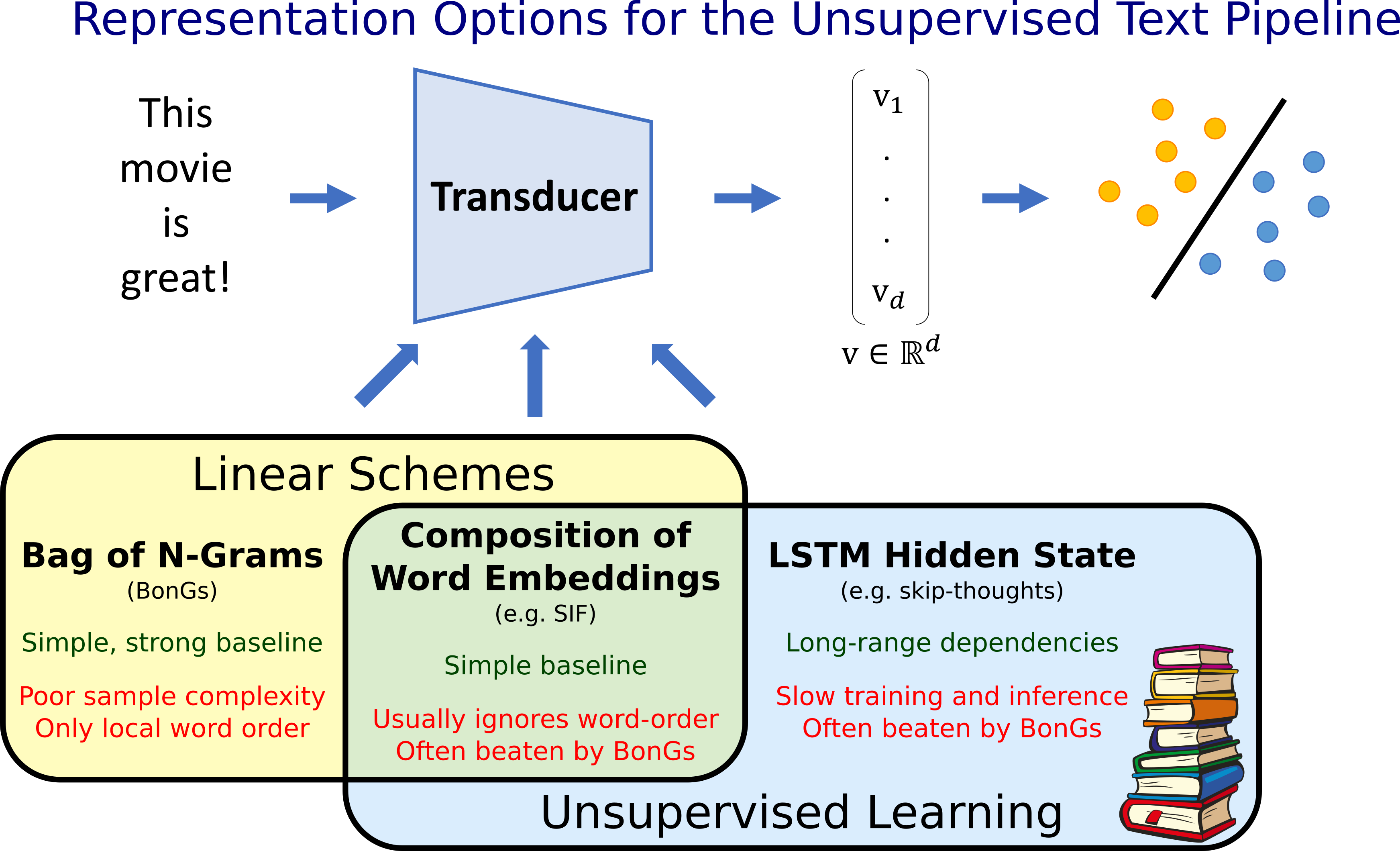## Incorporating local word order: $n$-gram embeddings

Bigrams are ordered word-pairs that appear in the sentence, and $n$-grams are ordered $n$-tuples. A document with $k$ words has $k-1$ bigrams and $k-n+1$ $n$-grams. The Bag of n-gram (BonG) representation of a document refers to a long vector whose each entry is indexed by all possible $n$-grams, and contains the number of times the corresponding $n$-gram appears in the document. Linear classifiers trained on BonG representations are a surprisingly strong baseline for document classification tasks. While $n$-grams don’t directly encode long-range dependencies in text, one hopes that a fair bit of such information is implicitly present.

A trivial idea for incorporating $n$-grams into SIF embeddings would be to treat $n$-grams like words, and compute word embeddings for them using either GloVe and word2vec. This runs into the difficulty that the number of distinct $n$-grams in the corpus gets very large even for $n=2$ (let alone $n=3$), making it almost impossible to solve word2vec or GloVe. Thus one gravitates towards a more compositional approach.

Compositional $n$-gram embedding: Represent $n$-gram $g=(w_1,\dots,w_n)$ as the element-wise product $v_g=v_{w_1}\odot\cdots\odot v_{w_n}$ of the embeddings of its constituent words.

Note that due to the element-wise multiplication we actually represent unordered $n$-gram information, not ordered $n$-grams (the performance for order-preserving methods is about the same). Now we are ready to define our Distributed Co-occurrence (DisC) embeddings.

The DisC embedding of a piece of text is just a concatenation for $(v_1, v_2, \ldots)$ where $v_n$ is the sum of the $n$-gram embeddings of all $n$-grams in the document (for $n=1$ this is just the sum of word embeddings).

Note that DisC embeddings leverage classic Bag-of-n-Gram information as well as the power of word embeddings. For instance, the sentences “Loved this movie!” and “I enjoyed the film.” share no $n$-gram information for any $n$, but their DisC embeddings are fairly similar. Thus if the first example comes with a label, it gives the learner some idea of how to classify the second. This can be useful especially in settings with few labeled examples; e.g. DisC outperform BonG on the Stanford Sentiment Treebank (SST) task, which has only 6,000 labeled examples. DisC embeddings also beat SIF and a standard LSTM-based method, Skipthoughts. On the much larger IMDB testbed, BonG still reigns at top (although DisC is not too far behind).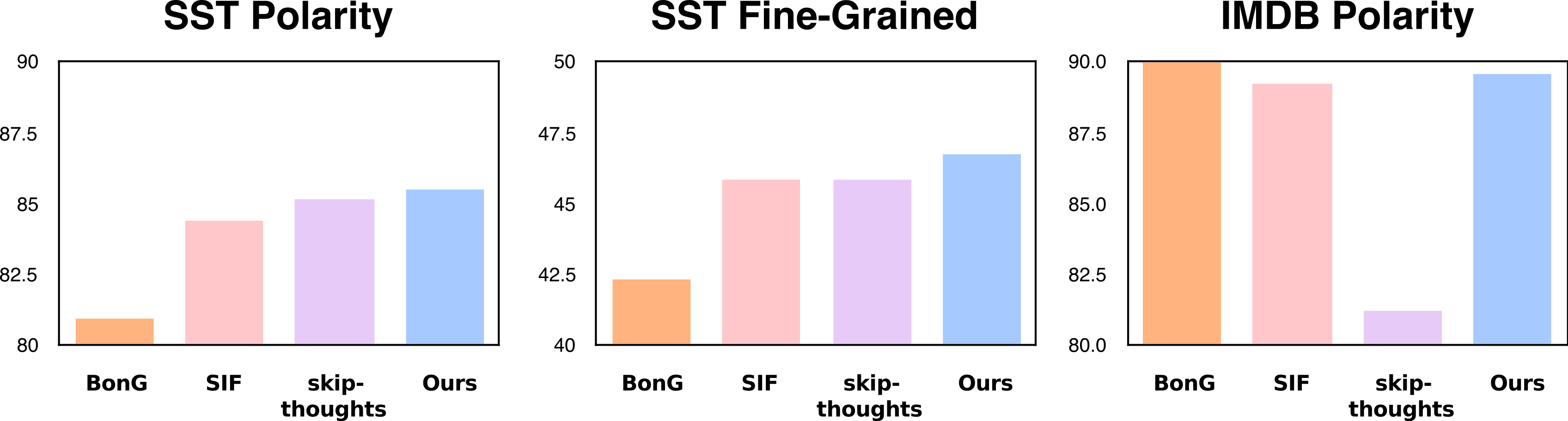Skip-thoughts does match or beat our DisC embeddings on some other classification tasks, but that’s still not too shabby an outcome for such a simple method. (By contrast, LSTM methods can take days or weeks of training, and are quite slow to evaluate at test time on a new piece of text.)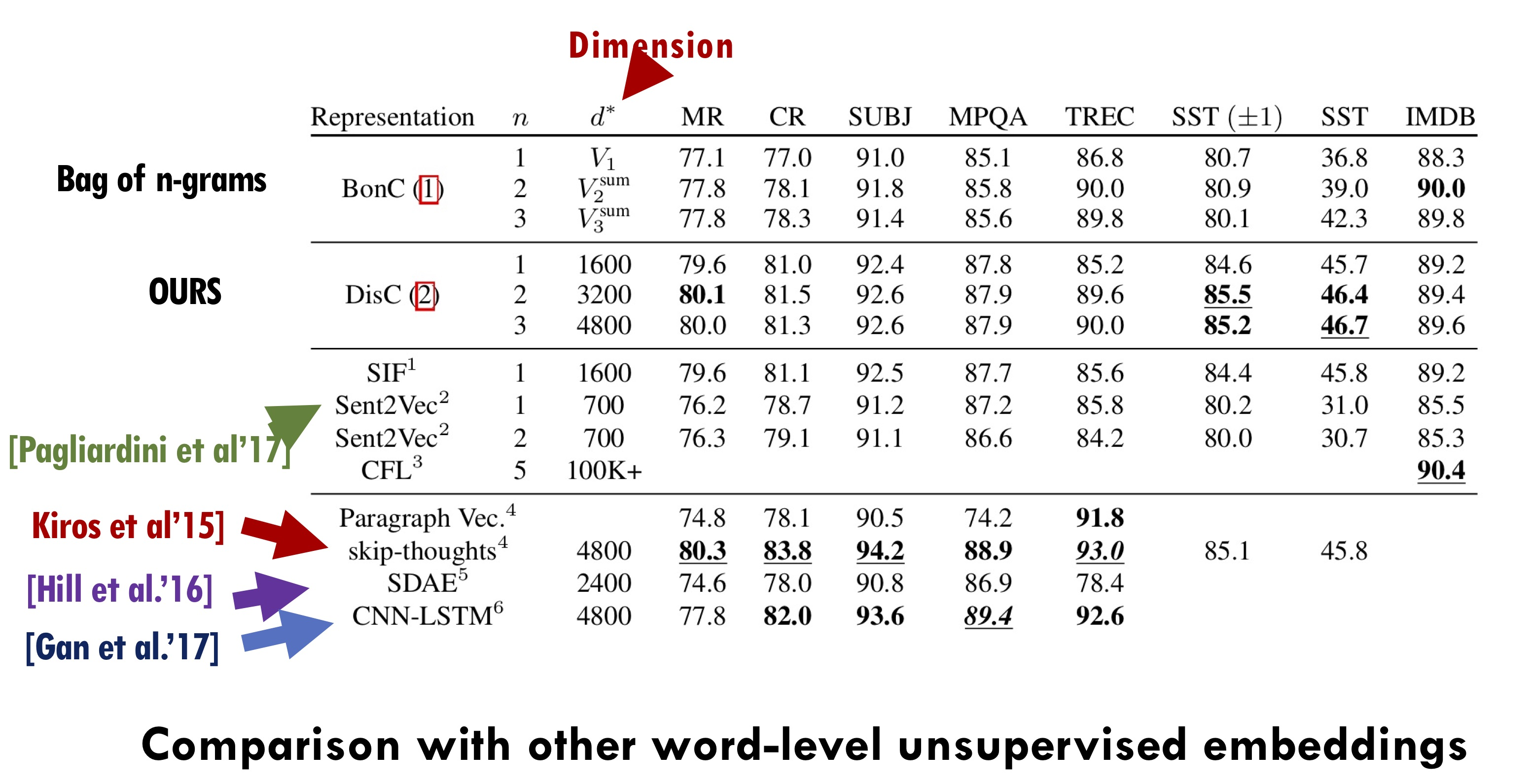## Some theoretical analysis via compressed sensing

A linear SIF-like embedding represents a document with Bag-of-Words vector $x$ as $$\sum_w \alpha_w x_w v_w,$$ where $v_w$ is the embedding of word $w$ and $\alpha_w$ is a scaling term. In other words, it represents document $x$ as $A x$ where $A$ is the matrix with as many columns as the number of words in the language, and the column corresponding to word $w$ is $\alpha_w A$. Note that $x$ has many zero coordinates corresponding to words that don’t occur in the document; in other words it’s a sparse vector.

The starting point of our DisC work was the realization that perhaps the reason SIF-like embeddings work reasonably well is that they preserve the Bag-of-words information, in the sense that it may be possible to easily recover $x$ from $A$. This is not an outlandish conjecture at all, because compressed sensing does exactly this when $x$ is suitably sparse and matrix $A$ has some nice properties such as RIP or incoherence. A classic example is when $A$ is a random matrix, which in our case corresponds to using random vectors as word embeddings. Thus one could try to use random word embeddings instead of GloVe vectors in the construction and see what happens! Indeed, we find that so long as we raise the dimension of the word embeddings, then text embeddings using random vectors do indeed converge to the performance of BonG representations.

This is a surprising result, as compressed sensing does not imply this per se, since the ability to reconstruct the BoW vector from its compressed version doesn’t directly imply that the compressed version gives the same performance as BoW on linear classification tasks. However, a result of Calderbank, Jafarpour, & Schapire shows that the compressed sensing condition that implies optimal recovery also implies good performance on linear classification under compression. Intuitively, this happens because of two facts.

$$\mbox{1) Optimum linear classifier c^* is convex combination of datapoints.} \quad c^* = \sum_{i}\alpha_i x_i.$$ $$\mbox{(2) RIP condition implies} <Ax, Ax'> \approx <x, x'>~\mbox{for k-sparse}~x, x'.$$

Furthermore, by extending these ideas to the $n$-gram case, we show that our DisC embeddings computed using random word vectors, which can be seen as a linear compression of the BonG representation, can do as well as the original BonG representation on linear classification tasks. To do this we prove that the “sensing” matrix $A$ corresponding to DisC embeddings satisfy the Restricted Isometry Property (RIP) introduced in the seminal paper of Candes & Tao. The theorem relies upon compressed sensing results for bounded orthonormal systems and says that then the performance of DisC embeddings on linear classification tasks approaches that of BonG vectors as we increase the dimension. Please see our paper for details of the proof.

It is worth noting that our idea of composing objects (words) represented by random vectors to embed structures ($n$-grams/documents) is closely related to ideas in neuroscience and neural coding proposed by Tony Plate and Pentti Kanerva. They also were interested in how these objects and structures could be recovered from the representations; we take the further step of relating the recoverability to performance on a downstream linear classification task. Text classification over compressed BonG vectors has been proposed before by Paskov, West, Mitchell, & Hastie, albeit with a more complicated compression that does not achieve a low-dimensional representation (dimension >100,000) due to the use of classical lossless algorithms rather than linear projection. Our work ties together these ideas of composition and compression into a simple text representation method with provable guarantees.

## A surprising lower bound on the power of LSTM-based text representations

The above result also leads to a new theorem about deep learning: text embeddings computed using low-memory LSTMs can do at least as well as BonG representations on downstream classification tasks. At first glance this result may seem uninteresting: surely it’s no surprise that the field’s latest and greatest method is at least as powerful as its oldest? But in practice, most papers on LSTM-based text embeddings make it a point to compare to performance of BonG baseline, and often are unable to improve upon that baseline! Thus empirically this new theorem had not been clear at all! (One reason could be that our theory requires the random embeddings to be somewhat higher dimensional than the LSTM work had considered.)

The new theorem follows from considering an LSTM that uses random vectors as word embeddings and computes the DisC embedding in one pass over the text. (For details see our appendix.)

We empirically tested the effect of dimensionality by measuring performance of DisC on IMDb sentiment classification. As our theory predicts, the accuracy of DisC using random word embeddings converges to that of BonGs as dimensionality increases. (In the figure below “Rademacher vectors” are those with entries drawn randomly from $\pm1$.) Interestingly we also find that DisC using pretrained word embeddings like GloVe reaches BonG performance at much smaller dimensions, an unsurprising but important point that we will discuss next.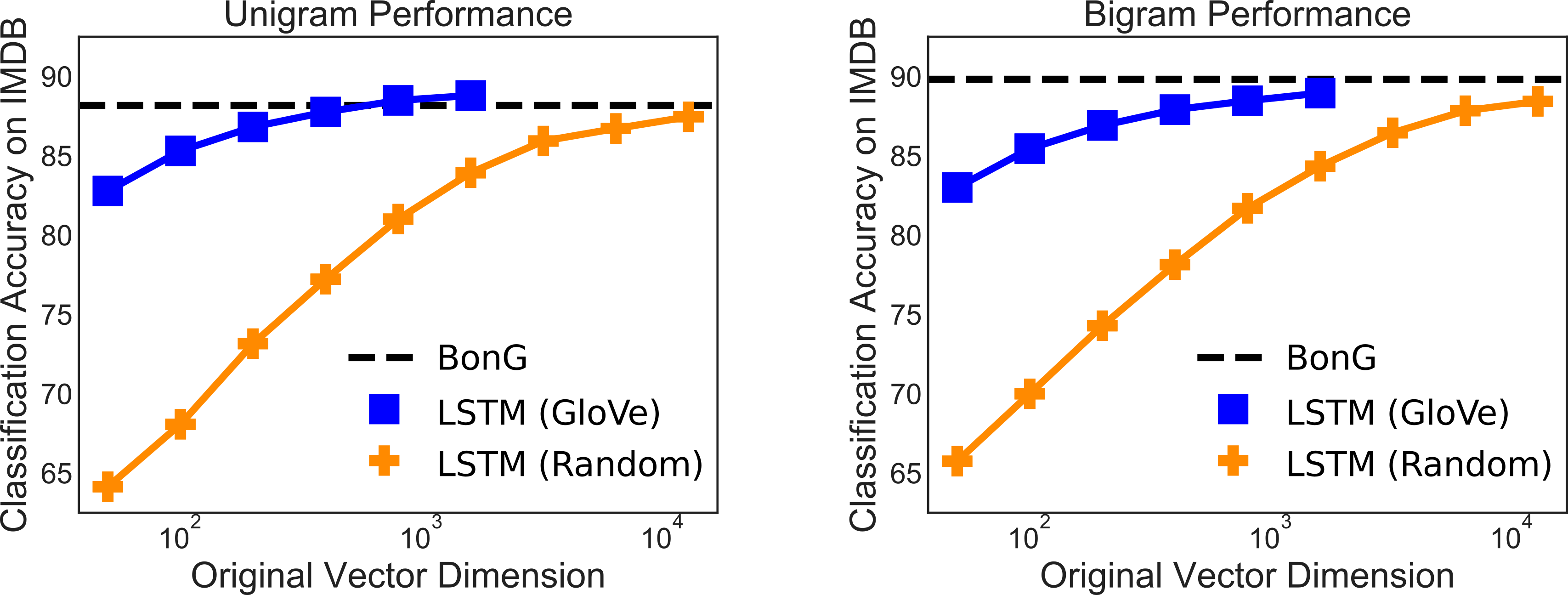## Unexplained mystery: higher performance of pretrained word embeddings

While compressed sensing theory is a good starting point for understanding the power of linear text embeddings, it leaves some mysteries. Using pre-trained embeddings (such as GloVe) in DisC gives higher performance than random embeddings, both in recovering the BonG information out of the text embedding, as well as in downstream tasks. However, pre-trained embeddings do not satisfy some of the nice properties assumed in compressed sensing theory such as RIP or incoherence, since those properties forbid pairs of words having similar embeddings.

Even though the matrix of embeddings does not satisfy these classical compressed sensing properties, we find that using Basis Pursuit, a sparse recovery approach related to LASSO with provable guarantees for RIP matrices, we can recover Bag-of-Words information better using GloVe-based text embeddings than from embeddings using random word vectors (measuring success via the $F_1$-score of the recovered words — higher is better).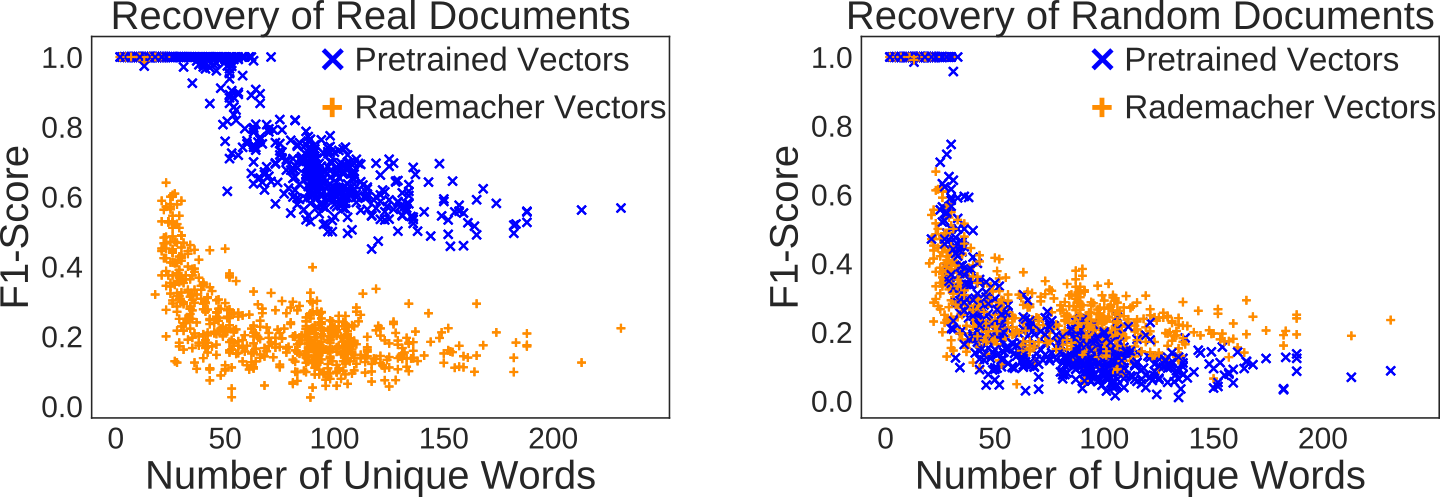Note that random embeddings are better than pretrained embeddings at recovering words from random word salad (the right-hand image). This suggests that pretrained embeddings are specialized — thanks to their training on a text corpus — to do well only on real text rather than a random collection of words. It would be nice to give a mathematical explanation for this phenomenon. We suspect that this should be possible using a result of Donoho & Tanner, which we use to show that words in a document can be recovered from the sum of word vectors if and only if there is a hyperplane containing the vectors for words in the document with the vectors for all other words on one side of it. Since co-occurring words will have similar embeddings, that should make it easier to find such a hyperplane separating words in a document from the rest of the words and hence would ensure good recovery.

However, even if this could be made more rigorous, it would only imply sparse recovery, not good performance on classification tasks. Perhaps assuming a generative model for text, like the RandWalk model discussed in an earlier post, could help move this theory forward.

## Discussion

Could we improve the performance of such simple embeddings even further? One promising idea is to define better $n$-gram embeddings than the simple compositional embeddings defined in DisC. An independent NAACL’18 paper of Pagliardini, Gupta, & Jaggi proposes a text embedding similar to DisC in which unigram and bigram embeddings are trained specifically to be added together to form sentence embeddings, also achieving good results, albeit not as good as DisC. (Of course, their training time is higher than ours.) In our upcoming ACL’18 paper with Yingyu Liang, Tengyu Ma, & Brandon Stewart we give a very simple and efficient method to induce embeddings for $n$-grams as well as other rare linguistic features that improves upon DisC and beats skipthought on several other benchmarks. This will be described in a future blog post.

Sample code for constructing and evaluating DisC embeddings is available, as well as solvers for recreating the sparse recovery results for word embeddings.